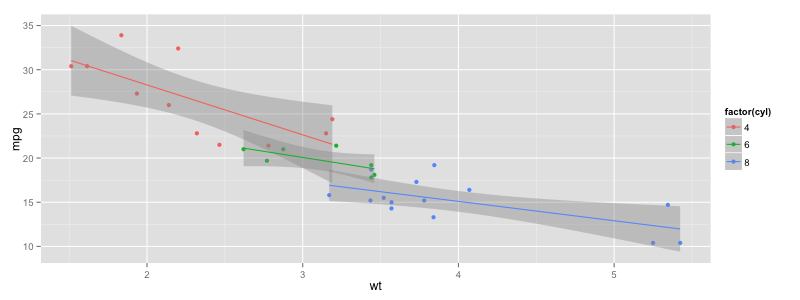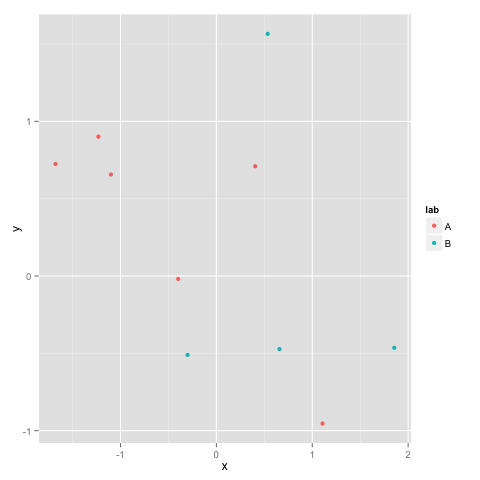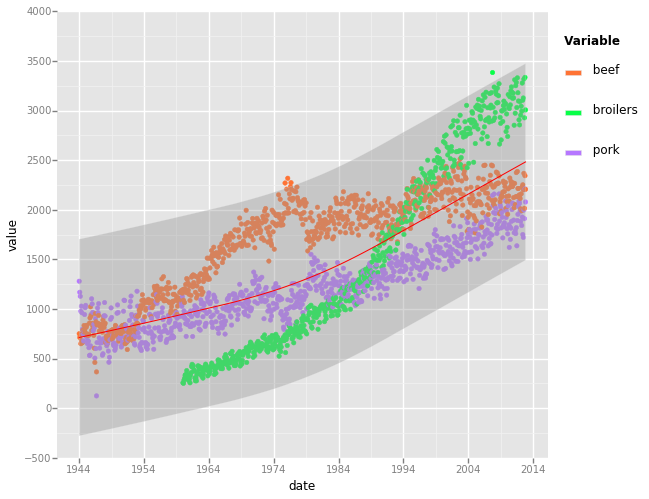# 在Python中调用ggplot的三种方法

更新时间：2015年04月08日 15:14:13   投稿：goldensun我要评论

RPy2

rpy2提供了对R语言的对象和方法的基本封装，当然也包括可视化的图库这一块。

```from rpy2 import robjects
from rpy2.robjects import Formula, Environment
from rpy2.robjects.vectors import IntVector, FloatVector
from rpy2.robjects.lib import grid
from rpy2.robjects.packages import importr, data
import rpy2.robjects.lib.ggplot2 as ggplot2

# The R 'print' function
rprint = robjects.globalenv.get("print")
stats = importr('stats')
grdevices = importr('grDevices')
base = importr('base')
datasets = importr('datasets')

mtcars = data(datasets).fetch('mtcars')['mtcars']

pp = ggplot2.ggplot(mtcars) + \
ggplot2.aes_string(x='wt', y='mpg', col='factor(cyl)') + \
ggplot2.geom_point() + \
ggplot2.geom_smooth(ggplot2.aes_string(group = 'cyl'),
method = 'lm')
pp.plot()
```

```%matplotlib inline

import uuid
from rpy2.robjects.packages import importr
from IPython.core.display import Image

grdevices = importr('grDevices')
def ggplot_notebook(gg, width = 800, height = 600):
fn = '{uuid}.png'.format(uuid = uuid.uuid4())
grdevices.png(fn, width = width, height = height)
gg.plot()
grdevices.dev_off()
return Image(filename=fn)
```RMagic

```%load_ext rmagic
library(ggplot2)
dat <- data.frame(x = rnorm(10), y = rnorm(10),
lab = sample(c('A', 'B'), 10, replace = TRUE))
x <- ggplot(dat, aes(x = x, y = y, color = lab)) + geom_point()
print(x)
```ggplot for python

ggplot是一个python的库，基本上是对R语言ggplot的功能移植到Python上。

```pip install ggplot

```

```%matplotlib inline
import pandas as pd
from ggplot import *
meat_lng = pd.melt(meat[['date', 'beef', 'pork', 'broilers']], id_vars='date')
ggplot(aes(x='date', y='value', colour='variable'), data=meat_lng) + \
geom_point() + \
stat_smooth(color='red')
```rpy2和Rmagic都是一种对R的桥接，所以都需要安装R。不同之处在于rpy2提供Python接口而Rmagic更接近R。

ggplot Python库是ggplot的Python移植，所以无需安装R，部署起来更为简单，但功能上也许和R的ggplot还有差距。